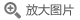## 常微分方程(第3版)(英文版)

• 定价： ￥79
• ISBN：9787519247645
• 开 本：24开 平装
• 作者：(俄罗斯)V.I.阿诺...
• 立即节省：
• 2018-08-01 第1版
• 2018-08-01 第1次印刷### 导语### 内容提要关于常微分方程方面的教科书有许多种，但本书却独具特物色，书中强调常微分方程的定性性质和几何性质及其它们的解，全书有272个几何插图，却没有一个复杂的数学公式。V.I.阿诺德著的《常微分方程(第3版)(英文版)》分为5章36节。本书是俄罗斯数学家(1937-2010)，1974年菲尔兹奖得主，他的许多优秀作品都被翻译为英文，本书是其中的一本，其简明的写作风格、严谨的数学基础结合物理直觉，给人一种很轻松漫谈式的教学特点，被评为最优秀的常微分教材。

### 目录

Chapter 1. Basic Concepts
1. Phase Spaces
1. Examples of Evolutionary Processes
2. Phase Spaces
3. The Integral Curves of a Direction Field
4. A Differential Equation and its Solutions
5. The Evolutionary Equation with a One-dimensional Phase Space
6. Example: The Equation of Normal Reproduction
7. Example: The Explosion Equation
8. Example: The Logistic Curve
9. Example: Harvest Quotas
10. Example: Harvesting with a Relative Quota
11. Equations with a Multidimensional Phase Space
12. Example: The Differential Equation of a Predator-Prey System
13. Example: A Free Particle on a Line
14. Example: Free Fall
15. Example: Small Oscillations
16. Example: The Mathematical Pendulum
17. Example: The Inverted Pendulum
18. Example: Small Oscillations of a Spherical Pendulum
2. Vector Fields on the Line
1. Existence and Uniqueness of Solutions
2. A Counterexample
3. Proof of Uniqueness
4. Direct Products
5. Examples of Direct Products
6. Equations with Separable Variables
7. An Example: The Lotka-Volterra Model
3. Linear Equations
1. Homogeneous Linear Equations
2. First-order Homogeneous Linear Equations with Periodic Coefficients
3. Inhomogeneous Linear Equations
4. The Influence Function and b-shaped Inhomogeneities
5. Inhomogeneous Linear Equations with Periodic Coefficients
4. Phase Flows
1. The Action of a Group on a Set
2. One-parameter Transformation Groups
3. One-parameter Diffeomorphism Groups
4. The Phase Velocity Vector Field
5. The Action of Diffeomorphisms on Vector Fields and Direction Fields
1. The Action of Smooth Mappings on Vectors
2. The Action of Diffeomorphisms on Vector Fields
3. Change of Variables in an Equation
4. The Action of a Diffeomorphism on a Direction Field
5. The Action of a Diffeomorphism on a Phase Flow
6. Symmetries
1. Symmetry Groups
2. Application of a One-parameter Symmetry Group to Integrate an Equation
3. Homogeneous Equations
4. Quasi-homogeneous Equations
5. Similarity and Dimensional Considerations
6. Methods of Integrating Differential Equations
Chapter 2. Basic Theorems
7. Rectification Theorems
1. Rectification of a Direction Field
2. Existence and Uniqueness Theorems
3. Theorems on Continuous and Differentiable Dependence of the Solutions on the Initial Condition
4. Transformation over the Time Interval from to to t
5. Theorems on Continuous and Differentiable Dependence on a Parameter
6. Extension Theorems
7. Rectification of a Vector Field
8. Applications to Equations of Higher Order than First
1. The Equivalence of an Equation of Order n and a System of n First-order Equations
2. Existence and Uniqueness Theorems
3. Differentiability and Extension Theorems
Chapter 3. Linear Systems
Chapter 4. Proofs of the Main Theorems
Chapter 5. Differential Equations on Manifolds
Examination Topics
Sample Examination Problems
Subject Index